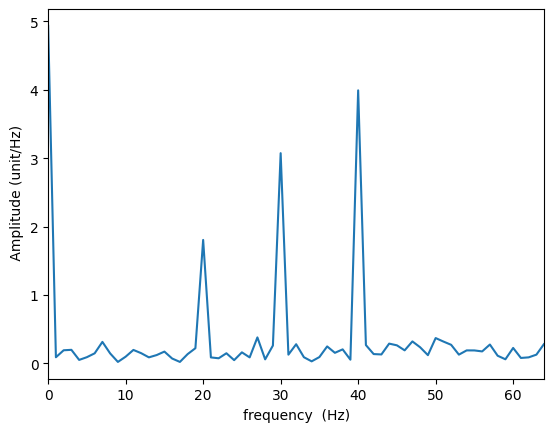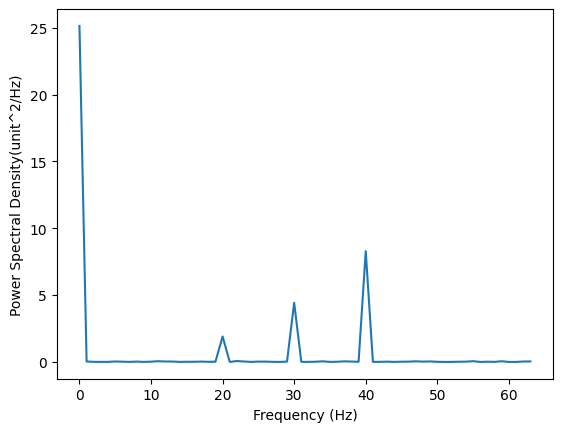# 傅里叶变换和功率谱密度



### 一 、离散傅里叶变换

$$X_k=\frac{T}{N}\sum^{N-1}_{n=0}x_n \exp(-2\pi ikn/N).$$

(下标和自变量的关系是$$t=n/N T，f=k/T, T$$ 为采样时长。)

import numpy as np
from scipy.fftpack import fft
import matplotlib.pyplot as plt
np.random.seed(2)

N = 128 # 采样点数
T=1 # 信号长度；信号长度整除周期时不会发生信号泄漏
dt=T/N # 采样间隔
Fs=N/T # 采样频率, 须大于两倍的最高频率
t=np.arange(0,T,dt)

signal=5+2*np.sin(2*np.pi*20*t)+3*np.sin(2*np.pi*30*t)+4*np.sin(2*np.pi*40*t)+np.random.randn(len(t))  # 采集的信号
fft_data = fft(signal)*dt # 快速傅立叶变换
fft_amp0 = np.array(np.abs(fft_data)*2)
fft_amp0=0.5*fft_amp0 # 0频率的幅值除以点数N，非零频率除以 N/2
list0 = np.array(range(0, N))
freq0 = list0/T # 频率坐标
plt.plot(freq0, fft_amp0)
plt.xlim(0,int(Fs/2))
plt.xlabel('frequency (Hz)')
plt.ylabel(' Amplitude (unit/Hz)')
plt.show()### 二、功率谱密度

Parseval 定理：总能量$$\mathrm{[unit^2\cdot s]} = \int_{-\infty}^{+\infty}|x(t)|^2\mathrm{d}t=\int_{-\infty}^{+\infty}|X(f)|^2\mathrm{d}f$$

### 三、功率谱密度的估计

#### 1.周期图法

$$S(f)=\frac{1}{N}|\sum^{N-1}_{n=0}x_n\exp{-2\pi ift}|^2,$$

Pxx = np.abs(fft(signal))**2/N/Fs*2 #单边谱，非零频率要乘2
Pxx = 0.5*Pxx
freq0 = Fs*list0/N

freqs = freq0[:len(signal)//2]
Pxx = Pxx[:len(signal)//2]

plt.plot(freqs, Pxx)
plt.xlabel('Frequency (Hz)')
plt.ylabel('Power Spectral Density(unit^2/Hz)')
plt.show()